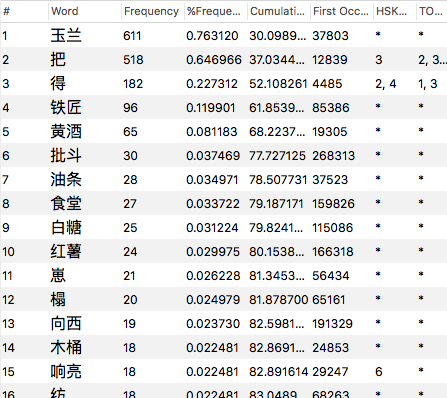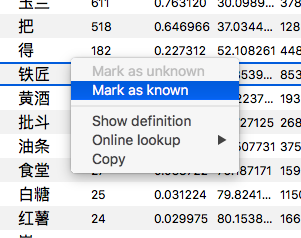# The Word List View¶

The word list view displays frequency and other statistics for each word of the currently open document.It is divided in to three lists:

• All - all the words in the document.
• Known - all the words in the document that you know (as determined by whether the word is in the current word list).
• Unknown - all the words in the document that you do not know (i.e. any word not in the current word list).

Clicking on the appropriate tab will let you view the different lists.

Multiple columns of information are displayed for each word, such as the word frequency and the HSK Level. You can click on the header of each column to sort the entire list by that column. Clicking the same header again will alternate between ascending and descending order.

The following is a full description of each column:

• Word - the word from the document.

• Frequency - the total number of times the word appeared in the document. For example, if you have a document with 1,000 words, and the word ‘的’ appears 30 times, then the frequency for ‘的’ would be 30.

• % Frequency - the same as `Frequency`, but expresssed as a percentage of the total words in the document. For example, if a word appeared 30 times and there were 1,000 words in the document, then that word would have a `% Frequency` of 3%.

• Cumulative % Frequency - the sum of all preceding `% Frequencies`, ordered from most frequent to least frequent.

For example, if the most frequent word has a `% Frequency` of 10% and the next most frequent word had a frequency of 5%, and the next most frequent word had a frequency of 3%, then those words would have `Cumulative % Frequencies` of 10%, 15% and 18% respectively (10 + 5 = 15, 15 + 3 = 18) and so on for every word in the document, up to 100%.

This figure lets you know that if you have learnt all the most frequent words up to a certain point, what percentage of words in the total document you will understand.

• First Occurrence - the position in the document where the word first appeared, expressed as a byte offset from the beginning of the file. The lower the number, the earlier this word appeared in the document. The higher the number, the later it appeared.

For example in the sentence ‘右边中间部分有一个表头’, ‘右边’ would have the lowest `First Occurrence` (because it appears first) and ‘表头’ would have the highest (because it appears last).

• HSK Level - the HSK level of this word, ranging from from 1-6, or * if the word is not defined in the HSK word lists.

If a word appears in multiple HSK levels, then multiple levels will be displayed, e.g. an HSK Level of `2, 4` would mean that the word appears in both the HSK 2 and the HSK 4 word lists.

• TOCFL Level - the TOCFL level of this word, ranging from from 1-5, or * if the word is not defined in the TOCFL word lists.

If a word appears in multiple TOCFL levels, then multiple levels will be displayed, e.g. a TOCFL Level of `2, 4` would mean that the word appears in both the TOCFL 2 and the TOCFL 4 word lists.

## Toggle Known State¶

You can toggle the known/unknown state of any word in the world list view by holding down `⌘` and double-clicking with the left mouse button.